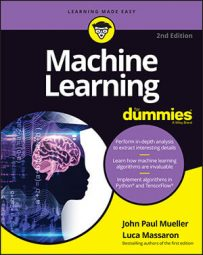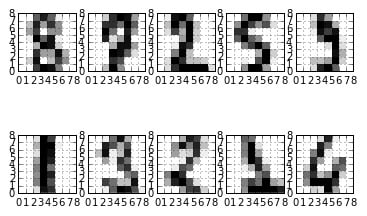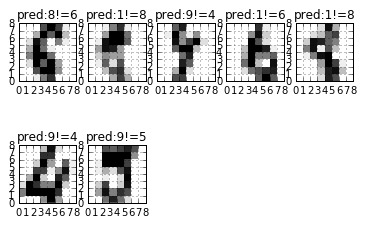##### Machine Learning For DummiesAs an example of how you can use an SVM to work out a complex problem for machine learning, here you find a demonstration of a handwritten recognition task and how to solve it using a nonlinear kernel, the RBF. The SVM algorithm learns from the digits dataset available from the module datasets in the Scikit-learn package. The digits dataset contains a series of 8-x-8 grayscale pixel images of handwritten numbers ranging from 0 to 9.

The problem is quite simple when compared to many problems that image recognition engines solve today, but it helps you grasp the potential of the learning approach. Even though the example relies on Python, you could create a similar example using R because both languages rely on the same LIBSVM library working behind the scenes. The example output appears below.

`import matplotlib.pyplot as plt`

`import matplotlib as mpl`

`from sklearn.datasets import load_digits`

`import numpy as np`

`import random`

` `

`digits = load_digits()`

`X,y = digits.data, digits.target`

` `

`%matplotlib inline`

`random_examples = [random.randint(0,len(digits.images))`

`for i in range(10)]`

`for n,number in enumerate(random_examples):`

`plt.subplot(2, 5, n+1)`

`plt.imshow(digits.images[number],cmap='binary',`

`interpolation='none', extent=[0,8,0,8])`

`plt.grid()`

`plt.show()`A sample from the MNIST handwritten digit dataset provided by Scikit-learn.

The example code randomly prints a few samples so that you can see an example of the different handwritten styles found in the dataset. Your printed examples may differ. The code renders the graphic information from a series of numbers, placed on a vector, each one pointing to a pixel in the image. The algorithm learns that if certain pixels activate together, the represented image corresponds to a particular number.

This example demonstrates the idea espoused by the Analogizers tribe: Certain patterns correspond to answers based on an analogy. SVMs tend to hide this fact because they seem to provide a linear combination of weighted features, just as linear regression does, but recall that when applying kernel functions, optimization is based on the dot products of examples.

Dot products are a way to estimate distance between points in order to divide the features space into homogeneous partitions having the same class inside. Distance is a way to establish similarities in a space made of features. Then SVMs works on similarity, associating points and patterns based on distance.

To verify the results from the model correctly, you first extract 30 percent of the examples to use as a test set (an out-of-sample). You also want to prepare the features by rescaling them in the range from –1 to +1 to ease the SVM computations. The algorithm first learns the parameters from the transformation of the training set alone and only after they are applied to the test set so that you avoid any kind of out-of-sample information leakage.

A critical action to take before feeding the data into an SVM is scaling. Scaling transforms all the values to the range between –1 to 1 (or from 0 to 1, if you prefer). Scaling transformation avoids the problem of having some variables influence the algorithm and makes the computations exact, smooth, and fast.

The code begins by adopting an SVM with a nonlinear kernel. To check the usefulness of the representation by the machine learning algorithm, the example uses the accuracy score (the percentage of correct guesses) as a measure of how good the model is).

`from sklearn.cross_validation import train_test_split`

`from sklearn.cross_validation import cross_val_score`

`from sklearn.preprocessing import MinMaxScaler`

`# we keep 30% random examples for test`

`X_train, X_test, y_train, y_test = train_test_split(X,`

`y, test_size=0.3, random_state=101)`

`# we scale the data in the range [-1,1]`

`scaling = MinMaxScaler(feature_range=(-1, 1)).fit(X_train)`

`X_train = scaling.transform(X_train)`

`X_test = scaling.transform(X_test)`

` `

`from sklearn.svm import SVC`

`svm = SVC()`

`cv_performance = cross_val_score(svm, X_train, y_train,`

`cv=10)`

`test_performance = svm.fit(X_train, y_train).score(X_test,`

`y_test)`

`print ('Cross-validation accuracy score: %0.3f,'`

`' test accuracy score: %0.3f'`

`% (np.mean(cv_performance),test_performance))`

` `

`Cross-validation accuracy score: 0.981,`

`test accuracy score: 0.985`

After having verified the cross-validation score using the default hyper-parameters, the code uses a systematic search to look for better settings that could provide a larger number of exact answers. During the search, the code tests different combinations of linear and RBF together with `C` and `gamma` parameters. (This example can require a long time to run.)

from sklearn.grid_search import GridSearchCV

`import numpy as np`

`learning_algo = SVC(kernel='linear', random_state=101)`

`search_space = [{'kernel': ['linear'],`

`'C': np.logspace(-3, 3, 7)},`

`{'kernel': ['rbf'],`

`'C':np.logspace(-3, 3, 7),`

`'gamma': np.logspace(-3, 2, 6)}]`

`gridsearch = GridSearchCV(learning_algo,`

`param_grid=search_space,`

`refit=True, cv=10)`

`gridsearch.fit(X_train,y_train)`

`print ('Best parameter: %s'`

`% str(gridsearch.best_params_))`

`cv_performance = gridsearch.best_score_`

`test_performance = gridsearch.score(X_test, y_test)`

`print ('Cross-validation accuracy score: %0.3f,'`

`' test accuracy score: %0.3f'`

`% (cv_performance,test_performance))`

` `

`Best parameter: {'kernel': 'rbf', 'C': 1.0,`

`'gamma': 0.10000000000000001}`

`Cross-validation accuracy score: 0.988,`

`test accuracy score: 0.987`

The computations may take a few minutes, after which the computer reports the best `kernel`, `C`, and `gamma` parameters, together with an improved CV score reaching almost 99 percent accuracy. The accuracy is high, indicating that the computer can almost distinguish all the different ways to write numbers from 0 to 9. As a final output, the code prints the numbers that the SVM wrongly predicted in the test set.

`prediction = gridsearch.predict(X_test)`

`wrong_prediction = (prediction!=y_test)`

`test_digits = scaling.inverse_transform(X_test)`

`for n,(number,yp,yt) in enumerate(zip(`

`scaling.inverse_transform(X_test)[wrong_prediction],`

`prediction[wrong_prediction],`

`y_test[wrong_prediction])):`

`plt.subplot(2, 5, n+1)`

`plt.imshow(number.reshape((8,8)),cmap='binary',`

`interpolation='none',`

`extent=[0,8,0,8])`

`plt.title('pred:'+str(yp)+"!="+str(yt))`

`plt.grid()`

`plt.show()`The handwritten numbers that the SVM with RBF kernel couldn’t guess correctly.

The numbers that the algorithm got wrong are particularly difficult to guess, and it’s no wonder that it couldn’t get them all right. Also remember that the dataset provided by Scikit-learn is just a portion of the real MNIST dataset. The full dataset consists of 60,000 training examples and 10,000 test examples. Using the same SVC algorithm and the same settings, the SVC can learn the original dataset, allowing the computer to read any handwritten number you present.